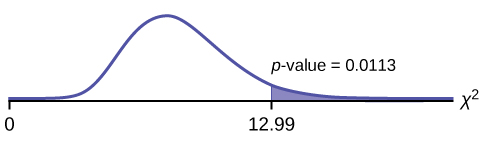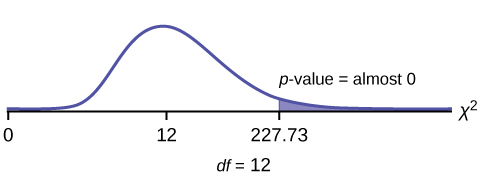## Test of Independence

### Learning Outcomes

• Conduct and interpret chi-square test of independence hypothesis tests

Tests of independence involve using a contingency table of observed (data) values.

The test statistic for a test of independence is similar to that of a goodness-of-fit test:

$\displaystyle{\sum_{(i\cdot{j})}}\frac{{({O}-{E})}^{{2}}}{{E}}$

where:

• O = observed values
• E = expected values
• i = the number of rows in the table
• j = the number of columns in the table

There are $\displaystyle{i}\cdot{j}$ terms of the form $\frac{{({O}-{E})}^{{2}}}{{E}}$.

A test of independence determines whether two factors are independent or not.

Note: The expected value for each cell needs to be at least five in order for you to use this test.

### Example

Suppose A = a speeding violation in the last year and B = a cell phone user while driving. If A and B are independent then P(A AND B) = P(A)P(B). A AND B is the event that a driver received a speeding violation last year and also used a cell phone while driving. Suppose, in a study of drivers who received speeding violations in the last year, and who used cell phone while driving, that 755 people were surveyed. Out of the 755, 70 had a speeding violation and 685 did not; 305 used cell phones while driving and 450 did not.

Let y = expected number of drivers who used a cell phone while driving and received speeding violations.

If A and B are independent, then P(A AND B) = P(A)P(B). By substitution,

$\displaystyle\frac{{y}}{{755}}={(\frac{{70}}{{755}})}{(\frac{{305}}{{755}})}$

Solve for
y: $\displaystyle{y}=\frac{{{({70})}{({305})}}}{{755}}={28.3}$

About 28 people from the sample are expected to use cell phones while driving and to receive speeding violations.

In a test of independence, we state the null and alternative hypotheses in words. Since the contingency table consists of two factors, the null hypothesis states that the factors are independent and the alternative hypothesis states that they are not independent (dependent). If we do a test of independence using the example, then the null hypothesis is:

H0: Being a cell phone user while driving and receiving a speeding violation are independent events.

If the null hypothesis were true, we would expect about 28 people to use cell phones while driving and to receive a speeding violation.

The test of independence is always right-tailed because of the calculation of the test statistic. If the expected and observed values are not close together, then the test statistic is very large and way out in the right tail of the chi-square curve, as it is in a goodness-of-fit.

The number of degrees of freedom for the test of independence is:

df = (number of columns – 1)(number of rows – 1)

The following formula calculates the expected number (E):

$\displaystyle{E}=\frac{{{(\text{row total})}{(\text{column total})}}}{\text{total number surveyed}}$

### try it

A sample of 300 students is taken. Of the students surveyed, 50 were music students, while 250 were not. Ninety-seven were on the honor roll, while 203 were not. If we assume being a music student and being on the honor roll are independent events, what is the expected number of music students who are also on the honor roll?

About 16 students are expected to be music students and on the honor roll.

### Example

In a volunteer group, adults 21 and older volunteer from one to nine hours each week to spend time with a disabled senior citizen. The program recruits among community college students, four-year college students, and nonstudents. The table below is a sample of the adult volunteers and the number of hours they volunteer per week.

Number of Hours Worked Per Week by Volunteer Type (Observed)The table contains observed (O) values (data).

Type of Volunteer 1–3 Hours 4–6 Hours 7–9 Hours Row Total
Community College Students 111 96 48 255
Four-Year College Students 96 133 61 290
Nonstudents 91 150 53 294
Column Total 298 379 162 839

Is the number of hours4 volunteered independent of the type of volunteer?

Solution:

The observed table and the question at the end of the problem, “Is the number of hours volunteered independent of the type of volunteer?” tell you this is a test of independence. The two factors are number of hours volunteered and type of volunteer. This test is always right-tailed.

H0: The number of hours volunteered is independent of the type of volunteer.

Ha: The number of hours volunteered is dependent on the type of volunteer.

The expected result are in the table below.

Number of Hours Worked Per Week by Volunteer Type (Expected)
The table contains expected (E) values (data)
Type of Volunteer 1–3 Hours 4–6 Hours 7–9 Hours
Community College Students 90.57 115.19 49.24
Four-Year College Students 103.00 131.00 56.00
Nonstudents 104.42 132.81 56.77

For example, the calculation for the expected frequency for the top left cell is

$\displaystyle{E}=\frac{{{(\text{row total})}{(\text{column total})}}}{\text{total number surveyed}}=\frac{{{({255})}{({298})}}}{{839}}={90.57}$

Calculate the test statistic:χ2 = 12.99 (calculator or computer)

Distribution for the test: $\displaystyle\chi^{2}_{4}$

Graph:Probability statement:p-value=P(χ2 > 12.99) = 0.0113

Compare α and the p-value: Since no α is given, assume α = 0.05. p-value = 0.0113. α > p-value.

Make a decision: Since α > p-value, reject H0. This means that the factors are not independent.

Conclusion: At a 5% level of significance, from the data, there is sufficient evidence to conclude that the number of hours volunteered and the type of volunteer are dependent on one another.

For the example in the table titled “Number of Hours Worked Per Week by Volunteer Type (Expected),” if there had been another type of volunteer, teenagers, what would the degrees of freedom be?

Press the MATRX key and arrow over to EDIT. Press 1:[A]. Press 3 ENTER 3 ENTER. Enter the table values by row from the table. Press ENTER after each. Press 2nd QUIT. PressSTAT and arrow over to TESTS. Arrow down to C:χ2-TEST. Press ENTER. You should see Observed:[A] and Expected:[B]. Arrow down to Calculate. Press ENTER. The test statistic is 12.9909 and the p-value = 0.0113. Do the procedure a second time, but arrow down to Draw instead of calculate.

### try it

The Bureau of Labor Statistics gathers data about employment in the United States. A sample is taken to calculate the number of U.S. citizens working in one of several industry sectors over time. The table below shows the results:

Industry Sector 2000 2010 2020 Total
Nonagriculture wage and salary 13,243 13,044 15,018 41,305
Goods-producing, excluding agriculture 2,457 1,771 1,950 6,178
Services-providing 10,786 11,273 13,068 35,127
Agriculture, forestry, fishing, and hunting 240 214 201 655
Nonagriculture self-employed and unpaid family worker 931 894 972 2,797
Secondary wage and salary jobs in agriculture and private household industries 14 11 11 36
Secondary jobs as a self-employed or unpaid family worker 196 144 152 492
Total 27,867 27,351 31,372 86,590

We want to know if the change in the number of jobs is independent of the change in years. State the null and alternative hypotheses and the degrees of freedom.

H0: The number of jobs is independent of the year.Ha: The number of jobs is dependent on the year.df = 12Press the MATRX key and arrow over to EDIT. Press 1:[A]. Press 3 ENTER 3 ENTER. Enter the table values by row. Press ENTER after each. Press 2nd QUIT. Press STAT and arrow over to TESTS. Arrow down to C:χ2-TEST. Press ENTER. You should see Observed:[A] and Expected:[B]. Arrow down to Calculate. Press ENTER. The test statistic is 227.73 and thep−value = 5.90E – 42 = 0. Do the procedure a second time but arrow down to Draw instead ofcalculate.

### Example

De Anza College is interested in the relationship between anxiety level and the need to succeed in school. A random sample of 400 students took a test that measured anxiety level and need to succeed in school. This table shows the results. De Anza College wants to know if anxiety level and need to succeed in school are independent events.

Need to Succeed in School vs. Anxiety Level
Need to Succeed in School High Anxiety Med-high Anxiety Medium Anxiety Med-low Anxiety Low Anxiety Row Total
High Need 35 42 53 15 10 155
Medium Need 18 48 63 33 31 193
Low Need 4 5 11 15 17 52
Column Total 57 95 127 63 58 400
1. How many high anxiety level students are expected to have a high need to succeed in school?
2. If the two variables are independent, how many students do you expect to have a low need to succeed in school and a med-low level of anxiety?
3. $\displaystyle{E}=\frac{(\text{row total})(\text{column total})}{\text{total surveyed}}$ = ________
4. The expected number of students who have a med-low anxiety level and a low need to succeed in school is about ________.

Solution:

1. The column total for a high anxiety level is 57. The row total for high need to succeed in school is 155. The sample size or total surveyed is 400.
$\displaystyle{E}=\frac{(\text{row total})(\text{column total})}{\text{total surveyed}}=\frac{155\cdot57}{400}=22.09$
The expected number of students who have a high anxiety level and a high need to succeed in school is about 22.
2. The column total for a med-low anxiety level is 63. The row total for a low need to succeed in school is 52. The sample size or total surveyed is 400.
3. $\displaystyle{E}=\frac{(\text{row total})(\text{column total})}{\text{total surveyed}} = 8.19$
4. 8

### try it

Refer back to the information in the Try It about the Bureau of Labor Statistics. How many service providing jobs are there expected to be in 2020? How many nonagriculture wage and salary jobs are there expected to be in 2020?

12,727, 14,965